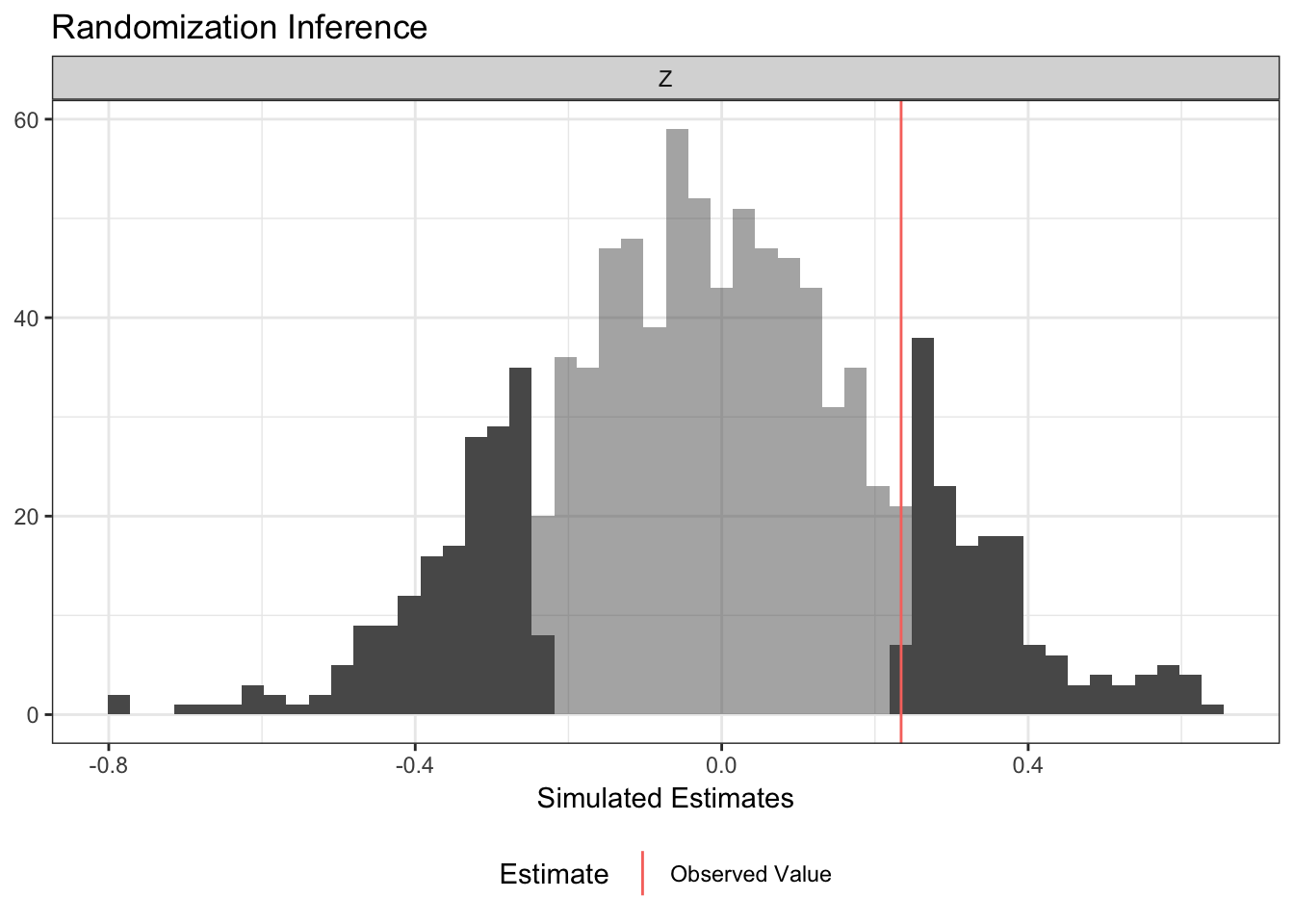ri2 makes conducting randomization inference easy and (with the blessing of the original authors) is the successor package to ri.

ri2 has specific support for the following:

1. All randomization schemes in randomizr.
2. Difference-in-means and OLS-adjusted estimates of ATE estimates using R-native formula syntax.
3. Multi-arm trials.
4. ANOVA-style hypothesis tests (e.g., testing interaction term under null of constant effects),

Additionally, ri2 provides:

1. Accommodation for arbitrary randomization schemes
2. Accommodation for arbitrary (scalar) test statistics

You can install ri2 is on CRAN

install.packages("ri2")

If you’d like to install the most current development release, you can use the following code:

install.packages("devtools")
devtools::install_github("acoppock/ri2")

Here is the basic syntax for a two-arm trial:

library(ri2)
#> Loading required package: randomizr
#> Loading required package: estimatr
N <- 100
declaration <- declare_ra(N = N, m = 50)

Z <- conduct_ra(declaration)
X <- rnorm(N)
Y <- .9 * X + .2 * Z + rnorm(N)
dat <- data.frame(Y, X, Z)

ri_out <-
conduct_ri(
formula = Y ~ Z,
declaration = declaration,
assignment = "Z",
sharp_hypothesis = 0,
data = dat
)

plot(ri_out)summary(ri_out)
#>   coefficient   estimate two_tailed_p_value null_ci_lower null_ci_upper
#> 1           Z -0.5887379              0.022    -0.4911486     0.4927481

The development of ri2 is supported by a Standards Grant from EGAP and the UK Department for International Development.